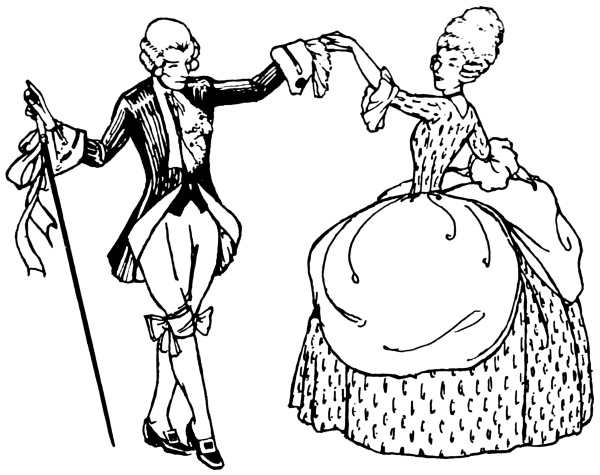# millis question .

Hello !

Can someone please give an example how to << convert>> 10 minutes in millis ?
thank a lot

10 minutes in milliseconds ?
You have a computer. Let it do the work for you

multiply milliseconds by 1000 to get seconds
multiply seconds by 60 to get minutes
multiply minutes by 10 to get 10 minutes

``````unsigned long tenMunutes = milliSeconds * 1000 * 60 * 10UL
``````

The UL specifier ensures that the calculation is not done as an int

Number of minuets X 60 to give the number of seconds

Then this X 1000 to give the number of milliseconds.

Or you could just multiply by 60,000, use a long int variable to do this as the int variables can’t hold a number that big.

You were quicker in the draw here BobYou were quicker in the draw here Bob

True, but I love your minuets !

UKHeliBob:
True, but I love your minuets !

Something like I you doYes dyslexia strikes again.UKHeliBob:
multiply milliseconds by 1000 to get seconds
multiply seconds by 60 to get minutes
multiply minutes by 10 to get 10 minutes

I think that needs to be reconsidered...R